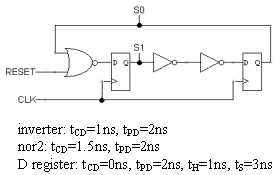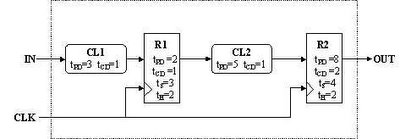Showing posts with label Sequential Circuits. Show all posts
Showing posts with label Sequential Circuits. Show all posts

## sequential circuit• What is the smallest clock period for which the circuit still operates correctly?
• By removing the pair of inverters and connecting the Q output of the left register directly to the D input of the right register, if the clock period could be adjusted appropriately, would the optimized circuit operate correctly? If so, explain the adjustment to the clock period that would be needed.
• When the RESET signal is set to "1" for several cycles, what values are loaded into the registers? (Give values for S0 and S1)
• Assuming the RESET signal has been set to "0" and will stay that way, what value will with the registers have after the next clock edge assuming the current values are S0=1 and S1=1?
• Now suppose there is skew in the CLK signal such that the rising edge of CLK always arrives at the left register exactly 1ns before it arrives at the right register. What is the smallest clock period for which the FSM still operates correctly?

## sequential circuit

Calculate the timing parameters (tS, tH, tCD, tPD, tCLK) for this system as a whole.